Difference between revisions of "Separation processes"

Title: Separation Processes

Authors: Nick Pinkerton, Karen Schmidt, and James Xamplas

Date Presented: February 9, 2014 /Date Revised: February 1, 2014

Introduction

Essentially all chemical processes require the presence of a separation stage. Most chemical plants comprise of a reactor surrounded by many separators. Separators have a countless number of jobs inside of a chemical plant. A separator can process raw materials prior to the reaction, remove incondensable gases, remove undesired side products, purify a product stream, recycle materials back into the process, and many other jobs that are essential to the process.

Chemical engineers must understand the science of separation and the variety of ways that separation can take place. There are many ways to perform a separation some of these including: distillation, absorption, stripping, and extraction. The science of separation revolves around the presence of two phases that are in contact and equilibrium .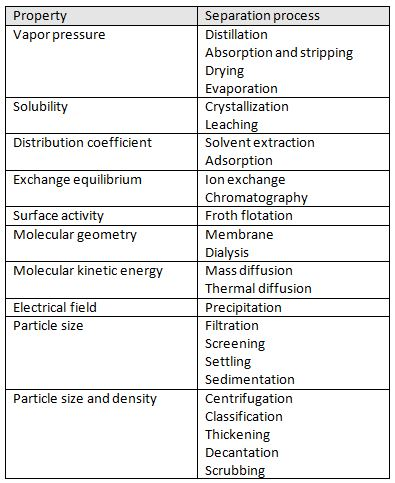Figure 1. Separation methods by property

Theory

Vapor-Liquid Equilibrium

Separation processes are based on the theory of vapor-liquid equilibrium. This theory states that streams leaving a stage in a separation process are in equilibrium with one another. The idea of equilibrium revolves around the idea that when there is vapor and liquid in contact with one another they are in constantly vaporizing and condensing. Different components in the mixture will condense and vaporize at different rates. There are three types of equilibrium conditions that can be subdivided into thermal, mechanical and chemical potential categories. These separate equilibrium states are given as: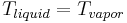$T_{liquid} = T_{vapor}$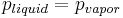$p_{liquid} = p_{vapor}$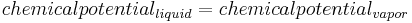$chemical potential_{liquid} = chemical potential_{vapor}$

Distillation

Flash Distillation

Flash Distillation is one of the simpler separation processes to be employed in a chemical plant. The main premise of flash distillation is that a portion of a liquid feed stream vaporizes in a flash chamber or a vapor feed condenses. Vapor-liquid equilibrium will cause the vapor phase and the liquid phase to have different compositions. The more volatile component of the mixture will compose of a larger portion of the vapor. This simple separation is easy to manufacture but does not result in large degrees of separation.

Flash distillation requires a feed stream that is pressurized and heated and then passed through a valve into a flash drum. The large pressure drop across the valve will result in a partial vaporization of the fluid. Vapor will be removed overhead from the flash drum while the remaining liquid will collect at the bottom of the drum and be removed. Most flash drums will contain an entrainment eliminator which is a screen that prevents liquid from being carried into the vapor effluent. Figure 2 shows a simple overview of the flash distillation process. As shown, there is a heater that flows into a let-down valve where the two-phase flow begins. Variables y and x are the mole fractions of the more volatile component in the vapor and liquid effluents, respectively.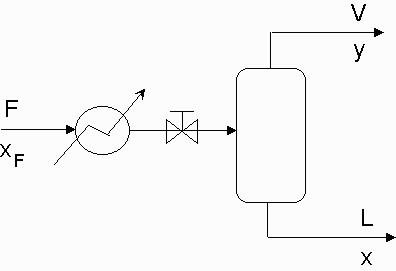Figure 2. Flash Distillation Flow Diagram

Column Distillation

Distillation columns are the most widely used separation technique used in the chemical industry, accounting for approximately 90% of all separations . Distillations in columns consist of multiple trays that each act at their own equilibrium conditions. Large columns are able to perform complete separations of binary mixtures as well as more complex multi-component mixtures.

Stages

Columns are separated into stages by the presence of trays. These trays allow for vapor-liquid contact and equilibrium to occur. Typically, the more stages in a column, the larger separation that can be achieved. There are many different types of trays that can be used in a column.

Sieve Trays

The simplest and least expensive tray type is the sieve tray which is a sheet of metal with holes punched into it to allow vapor flow. Sieve trays can have different hole patterns and sizes that will affect the tray efficiency and flow rates.

Bubble-Cap Trays

Bubble-cap trays consist of a weir around each hole in the tray which is covered with a cap that has holes or slots to allow vapor passage. Entrainment is about three times larger than a sieve tray. Bubble-cap trays require larger tray spacing than sieve tray design. Bubble-cap trays have been known to have problems with coking, polymer formation, or high fouling mixtures. Recently, very few new bubble-cap columns are being built due to the expense and marginal benefits. However, engineers will likely encounter bubble-cap columns still currently in operation.

Flow Patterns

Cross flow columns are the most common pattern for distillation columns. For liquid flows between 50 and 500 Gal/min, a cross flow column is appropriate. When liquid flow is increased above 500 Gal/min, an engineer should consider designing a double pass or multi-pass column. This will reduce the liquid gradient on the tray and reduce the downcomer loading .

Column Sizing

Column height will be dependent on the amount of trays required and the spacing between the trays. Normally, tray spacing of 0.15 m to 1 m is used. For columns, above 1 meter in diameter, 0.5 m can be used as an initial estimate.

Column diameter is influenced by the vapor flow rate in the column. The trays can not have excess liquid entrainment or high pressure drops; therefore, vapor velocity in the column must be maintained at a reasonable level.

An equation based on the Souders and Brown equation can be used as an estimate for the max allowable superficial vapor velocity,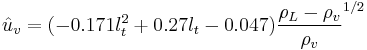$\hat u_v = (-0.171l_t^2 + 0.27l_t - 0.047){\frac{\rho_L - \rho_v}{\rho_v}}^{1/2}$

where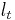$l_t$ is the plate spacing in meters,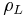$\rho_L$ is the density of the liquid stream, and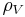$\rho_V$ is the density of the vapor stream.

Column diameter,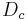$D_c$, can then be estimated using the relation,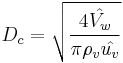$D_c = \sqrt{\frac{4\hat{V_w}}{\pi\rho_v\hat{u_v}}}$

where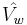$\hat{V_w}$ is the maximum vapor rate in kg/s .

Distillation Applications

Distillation is a process that can be implemented in various scales. There is both laboratory scaled distillation as well as very large industrial distillation. Other applications for distillation include food/alcohol processing and herb distillation for the perfume and medical industries. Typically laboratory scaled distillation occurs in batches whereas industrial distillation (e.g. fractional distillation of crude oil) occurs continuous with a constant distillate and bottom effluent streams.

Some applications of distillation are concerned the top stream only, some the bottom stream only and others both streams can be used for future products. In alcohol distillation for example, the water that is separated from the ethanol/water binary solution is discarded as waste water. In fractional distillation of crude oils, the heavy hydrocarbons at the bottom of the column are collected and sold along with the light hydrocarbons that appear in higher side draws .

Example Case: Ideal Distillation

Assume an equimolar mixture flowing at 10 mol/s of 20 mol% n-pentane, 30 mol% n-hexane, and 50 mol% n-heptane. Separate the mixture into 3 products: 99% pure n-pentane, 99% pure n-hexane, 99% n-heptane. Assume the feed and products are all liquids at the bubble points. There are two process alternatives to consider in this example. The direct sequence removes the most volatile species, pentane, in the first column, and then separates hexane and heptane in the second column. The indirect sequence separates the heaviest product, heptane, and then separates pentane from hexane in the second column. This example will consider the direct sequence. Next, we must decide if these species exhibit fairly ideal behavior during distillation. Since the n-alkanes have very similar properties, it is safe to assume they will display close to ideal behavior. The next step is to look up the boiling points of the 3 species. In this case, the normal boiling points of pentane, hexane, and heptane are 309 K, 342 K, and 372 K, respectively. Also, it is a good idea to look up relative volatilites, to further verify near-ideality of the mixture, but also to obtain the information necessary for the Underwood method, which we will employ to obtain a solution. The next step is to write out material balances based on molar flows and the design specifications. They go as follows:$\mu_I(nC5) + \mu_{II}(nC5) = 2 mol/s$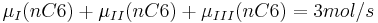$\mu_I(nC6) + \mu_{II}(nC6) + \mu_{III}(nC6) = 3 mol/s$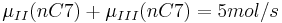$\mu_{II}(nC7) + \mu_{III}(nC7) = 5 mol/s$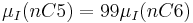$\mu_I(nC5) = 99\mu_I(nC6)$$\mu_{II}(nC5) = (5/990)\mu_{II}(nC6)$$\mu_{II}(nC7) = (5/990)\mu_{II}(nC6)$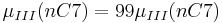$\mu_{III}(nC7) = 99\mu_{III}(nC7)$

where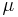$\mu$ represents the molar flow, and the subscript represents the product stream.

Solving this system of equations: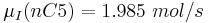$\mu_I(nC5) = 1.985\ mol/s$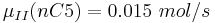$\mu_{II}(nC5) = 0.015\ mol/s$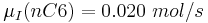$\mu_I(nC6) = 0.020\ mol/s$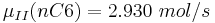$\mu_{II}(nC6) = 2.930\ mol/s$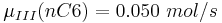$\mu_{III}(nC6) = 0.050\ mol/s$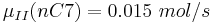$\mu_{II}(nC7) = 0.015\ mol/s$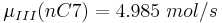$\mu_{III}(nC7) = 4.985\ mol/s$

At this point we have enough information to use Underwood's method to estimate the minimum vapor flows in the column. The following three equations are used in Underwood's method: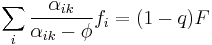$\sum_i \frac{\alpha_{ik}}{\alpha_{ik}-\phi}f_i = (1-q)F$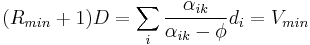$(R_{min}+1)D = \sum_i \frac{\alpha_{ik}}{\alpha_{ik}-\phi}d_i = V_{min}$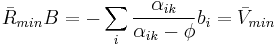$\bar R_{min}B = -\sum_i \frac{\alpha_{ik}}{\alpha_{ik}-\phi}b_i = \bar V_{min}$

where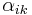$\alpha_{ik}$ is the relative volatility of species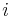$i$ to species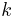$k$,$f_i$ the molar flow of species$i$ in the feed,$q$ the fraction of the feed that joins the liquid stream at the feed tray,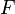$F$ the total molar flow of the feed,$D$ the molar flow of the distillate,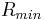$R_{min}$ the minimum reflux ratio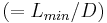$(=L_{min}/D)$,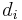$d_i$ the molar flow of species$i$ in the distillate,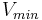$V_{min}$ the minimum vapor flow possible in the top section of the column to accomplish the desired separation,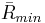$\bar R_{min}$ the minimum reboil ratio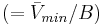$(=\bar V_{min}/B)$,$b_i$ the molar flow of species$i$ in the bottoms product, and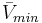$\bar V_{min}$ the minimum vapor flow in the bottom section of the column. The final variable,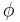$\phi$, will be solved for using the first Underwood equation, and it's value will be decided based on the relative volatilities of the key components in the column.

So, after solving the first Underwood equation, we get two values for$\phi$, 3.806 and 1.462. Because 3.806 is between the relative volatilities of the key components, we will substitute that value for$\phi$ into the second Underwood equation. Doing so for both columns gives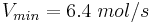$V_{min} = 6.4\ mol/s$ for the first column and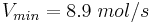$V_{min} = 8.9\ mol/s$ for the second column, for a total minimum vapor flow of 15.3 mol/s. The process would then be repeated for the indirect sequence, and the decision for which process to use would be justified by the process with the overall minimum vapor flow.

Absorption

An alternative to distillation for separating solutes from gas streams is absorption. The gas mixture comes into contact with a liquid solvent that readily absorbs the undesirable components from the gas stream, purifying the gas stream. This separation process is determined by the inputs of the liquid flow rate, temperature, and pressure.

The absorption factor, which can be determined mathematically, determines how readily a component will absorb in the liquid phase. The absorption factor of component i is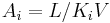$A_i=L/K_iV$

where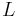$L$ is the liquid flow rate entering the column,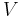$V$ is the vapor flow rate entering the column, and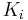$K_i$ is the vapor/liquid equilibrium ratio for component i . Higher absorption factors result in higher absorptivity into the liquid and a decrease in the number of trays required for separation, however a diminishing return occurs after the absorption factor is greater than 2.0. An absorption factor of 1.4 is most commonly used.

An industrial example is lean oil absorption, which is used to separate nitrogen and other impurities from natural gas. A lean oil is contacted with low quality natural gas, and the methane is selectively absorbed by the lean oil, leaving the impurities behind. The methane is subsequently regenerated from the rich oil as high quality natural gas .

Stripping

This process separates solutes from solvents (often after absorption, to purify the solvent so that it can be recycled to an absorber). Stripping will depend on the vapor and liquid flow rates, as well as the temperature and pressure of the column. There is a temperature drop down the column, so columns generally have either an increased operating temperature or decreased operating pressure.

The stripping factor of component i is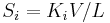$S_i=K_iV/L$

where$K_i$ is the vapor/liquid equilibrium ratio,$V$ is the vapor flow rate entering the column, and$L$ is the liquid flow rate entering the column, will determine how much of solute i will be stripped from the liquid into the vapor phase . The usual range for the stripping factor is between 1.2 and 2.0, with a stripping factor of 1.4 being most economic.

An example of stripping in industry is the deodorization of food items such as oils. The oil is heated and allowed to trickle down the column while steam flows up from the bottom of the column. At the vapor-liquid interface, volatile components of the oil transfer to the steam and are carried off the top of the column, leaving a purified oil product .

Other Separation Processes

Extraction

Liquid-liquid extraction is a process for components with overlapping boiling points and azeotropes. The process requires a solvent such that some of the components of the mixture are soluble, and then the components will be separated based on this solubility in the liquid. This process can operate at moderate temperatures and pressures, so is not very energy intensive. However, a distillation column is required to extract the solvent for recycle. More recently, supercritical fluids have replaced liquid solvents in some processes for L/L extraction, due to the solute’s ability to more rapidly diffuse through them. The issue with these fluids, however, is that they must be operated at extremely high pressures and temperatures, increasing both capital and operating expenses of the process .

Crystallization

This process recovers solutes that have been dissolved in solution. The resulting product is in the solid phase. Depending on the material properties of the solute and solvent, the solute is recovered by precipitation after cooling, removal of solvent, or adding precipitating agents. Crystallizers are designed based on phase equilibria, solubilities, rates and amounts of nuclei generated, and rates of crystal growth. Every crystallization process is a unique system, so plant evaluation is usually required before complete implementation. Crystallization can be performed in both batch and continuous processes, and design features can control crystal size to an extent .

Membrane Separation

This separations process uses selectively permeable membranes to separate components in a mixture. Typically, one of the components will freely pass through the barrier while the other components will not. The stream that passes through the membrane is the permeate and the stream that does not pass is the retentate. The driving force behind this separation is a pressure gradient. Membrane separation is beneficial because it can separate mixtures at the molecular and small particle level. Furthermore, there is no phase change required so the energy input is low. Limitations of this process include achieving high product purity, incompatibility with certain stream components, low operating temperature, and low flow rates. Although membrane separation is generally not scaled up, examples of scaled-up membrane separation include seawater desalination and hydrogen recovery .

Adsorption involves an adsorbent and adsorbate. The adsorbent is typically a solid, and will typically separate the adsorbate from the stream. This process usually includes a desorption step that regenerates the adsorbent for further use. Raising the temperature or increasing the concentration of the adsorbate can reverse the adsorption process. Although the recycle of the adsorbent is a very economic design feature, the downside of this step is that it results in a cyclic process, which introduces complexity to the overall process. Industrial applications of this process are for bulk separations and gas purification. The adsorption/desorption process in these situations involves a large amount of heat transfer, which design engineers must take into account when sizing and selecting equipment material .

These separations use external force fields or temperature gradients to separate responsive molecules or ions. The use of these processes is fairly limited to a few specialized industrial applications .

Settling and Sedimentation

In settling processes, solid particles or liquid drops are separated from a stream by gravity. The stream can be in either the liquid or gas phase. For vapor-liquid mixtures, flash drums are generally used to separate the mixture. The velocity of the vapor must be less than the settling velocity of the liquid drops for this separation to occur. For liquid-liquid separation, the horizontal velocity of the fluid must be low enough to allow the low-density droplets to rise to the interface and the high-density droplets to move away from the interface and coalesce. In sedimentation, the result of the process is a more concentrated slurry. Typically a flocculating agent is used to aid in the settling process. One way to perform this separation is to use a cone-shaped tank with a slowly revolving rake that scrapes and moves the thickened slurry to the center of the cone for removal .

Flotation

Flotation is a process designed for specific solid-solid mixtures. It works by generating gas bubbles in a liquid that attach to selected solid particle. Afterwards, the particles rise to the liquid surface where they are removed by an overflow weir or mechanical scraper. The separation depends on the surface properties of the particles and its preference to attach to the gas bubbles. To meet the necessary requirements of the flotation process, a number of additives can be used to control things like the pH of the liquid-solid mixture, the activity of the solid surface, and the froth that can assist in separation. The bubbles can be produced by gaseous dispersion, dissolution, or electrolysis of the liquid .

Centrifugation

This process is similar to external field separation in that an external force field is applied to separate a mixture. When gravity separation is too slow due to particle densities, particle size, settling velocity, or the formation of an emulsion, centrifugation is commonly used. Centrifugal force increases the total force acting on the particle and results in faster separation times. This process is generally used to separate solids from liquids, however it can also be used to separate two liquids with very different densities .

Drying

Drying is performed to remove liquid from a liquid-solid mixture and produce a dry solid. Water is most often the liquid removed, but organic liquids are removed from solids on occasion as well. The heat required to vaporize the liquid is usually obtained by a series of gas-solid contacting devices. Feed condition and temperature sensitivity of the solid dictate the type of contacting device that is used. There are two groups of dryers that differ by the dependence of either mechanical means or fluid motion for gas solid contact. Another feature of dryers is to use either direct (hot gas) or indirect (conductive surface) heating .

Evaporation

Evaporators separate solvents from a solution by evaporation. The difference between evaporation and distillation is that evaporation requires the solute be nonvolatile. Because of this, a high separation can be achieved with one stage. Evaporators are essentially reboilers, so evaporation is a very energy-intensive process with a high thermal economy .

Filtration

Filtration is a process that separates a mixture of solid in a liquid or gas by passing the mixture through a porous medium in which the particles do not pass. Filtration is done by either cake filtration (particles found on the surface of the filter) or depth filtration (particles found within the filter). Cake filtration is generally performed with a cloth as the filtration medium .